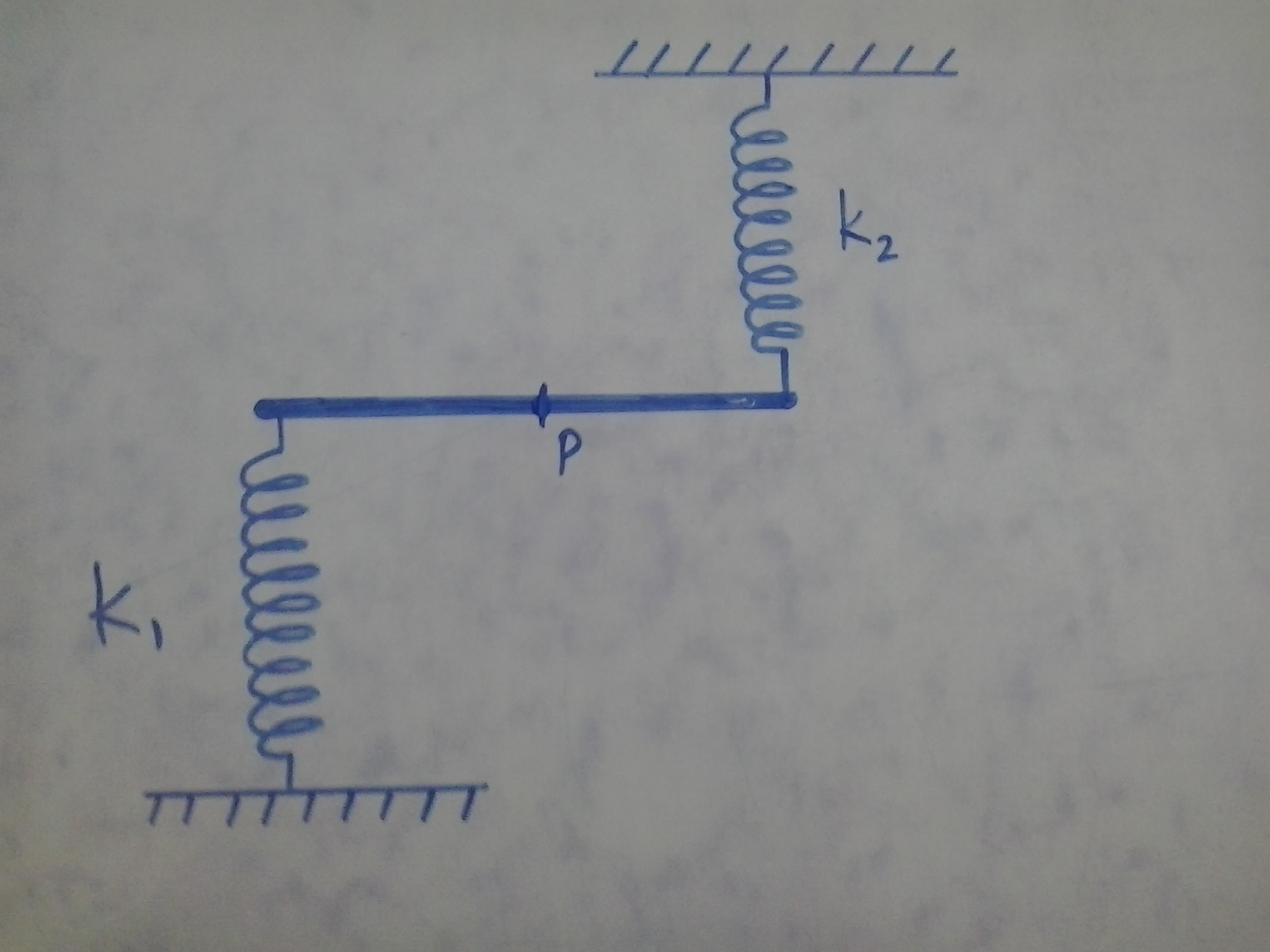# Hanging SHMA system consisting of a rod of mass $M$ and length $l$ is pivoted at its centre $P$. Two springs of spring constants $k_1$ and $k_2$ respectively are attached as shown. They are relaxed, when the rod is horizontal. What is the time period of the rod if it is given slight angular displacement.

Find $1000T$, if $T$ is the time period.

Details:

• $M = 10$ kg
• $k_1 = 100$ N/m
• $k_2 = 200$ N/m
×